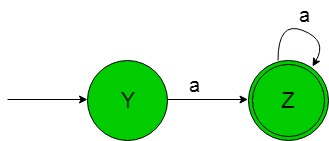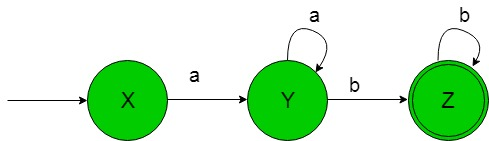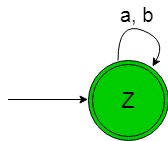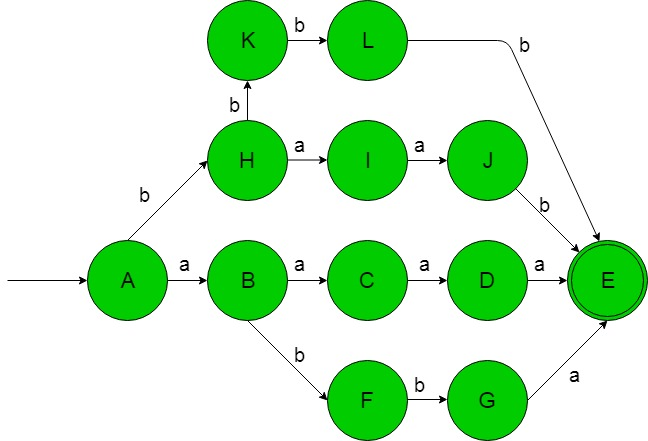# Designing Finite Automata from Regular Expression (Set 8)

• Last Updated : 20 Nov, 2019

In the below article, we shall see some Designing of Finite Automata form the given Regular Expression-

Regular Expression 1: Regular language,

`L1 = {an | n≥ 1}  `

The language of the given RE is-

`{a, aa, aaa, ..........}`

Its finite automata will be like below-In the above transition diagram, as we can see that initial state ‘Y’ on getting ‘a’ as the input it transits to a final state ‘Z’ and so on for the remaining states. Thus this FA accepting all the strings of the given RE language.

Regular Expression 2: Regular language,

`L2 = {anbm | n, m≥ 1}`

.
The language of the given RE is-

`{ab, aab, abb, aaaabb, ..........}`

Its finite automata will be like below-In the above transition diagram, as we can see that initial state ‘X’ on getting ‘a’ as the input it transits to a
state ‘Y’ and so on for the remaining states. Thus this FA accepting all the strings of the given RE language.

Regular Expression 3: Regular language,

`L3 = (a+b)*  `

The language of the given RE is-

`{ε, a, aa, aaa, aabbb, ........} `

Its finite automata will be like below-In the above transition diagram, as we can see that initial and final state ‘Z’ on getting either ‘a’ or ‘b’ as the input it remains in the state of itself. Thus this FA accepting all the strings of the given RE language.

Note: The below REs are equivalent to each other-

```= (a+b)*
= (a*+b*)*
= (a*b*)*
= (a*+b)*
= (a+b*)*
= a*(ba*)*
= b*(ab*)* ```

Regular Expression 4: Regular language,

`L4 = {wwR | |w|=2, Σ={a, b}*} `

The language of the given RE is-

`{aaaa, abba, baab, bbbb}`

Its finite automata will be like below-In the above transition diagram, as we can see that initial state ‘A’ on getting ‘a’ as the input it transits to a state ‘b’ and on getting ‘b’ as the input it transits to a state ‘H’ and so on for the remaining states. Thus this FA accepting all the strings of the given RE language.

Note: Below expression is not a Regular expression because length of the string ‘w’ is not bounded.

`{wwR | Σ={a, b}*}`
My Personal Notes arrow_drop_up
Recommended Articles
Page :